Courses

# Linear Transform MCQ - 4

## 20 Questions MCQ Test Topic-wise Tests & Solved Examples for IIT JAM Mathematics | Linear Transform MCQ - 4

Description
This mock test of Linear Transform MCQ - 4 for Mathematics helps you for every Mathematics entrance exam. This contains 20 Multiple Choice Questions for Mathematics Linear Transform MCQ - 4 (mcq) to study with solutions a complete question bank. The solved questions answers in this Linear Transform MCQ - 4 quiz give you a good mix of easy questions and tough questions. Mathematics students definitely take this Linear Transform MCQ - 4 exercise for a better result in the exam. You can find other Linear Transform MCQ - 4 extra questions, long questions & short questions for Mathematics on EduRev as well by searching above.
QUESTION: 1

### Which one of the following is an eigenvector of the matrix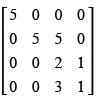Solution: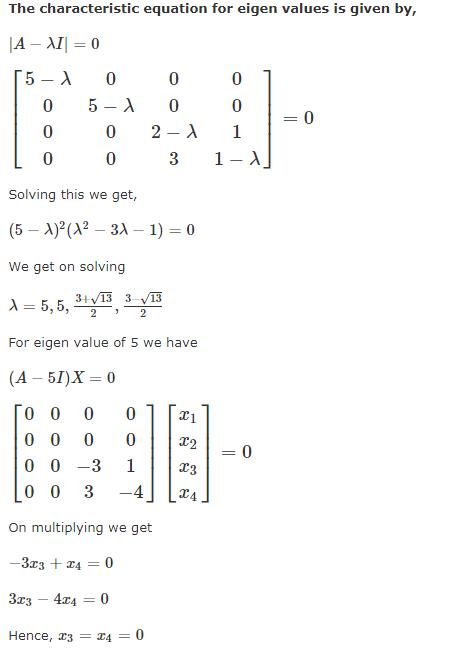So option (a) only satisfys the condition

QUESTION: 2

Solution:
QUESTION: 3

### An eigenvector of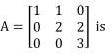Solution:
QUESTION: 4

The eigenvalues of the matrix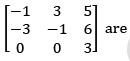Solution:
QUESTION: 5

For the matrix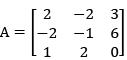one of the eigenvalues is 3. The other two eigenvalues are

Solution:
QUESTION: 6

The characteristic vector of the matrix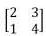corresponding to characteristic root 1 is

Solution:
QUESTION: 7

The eigenvalues of a skew symmetric matrix are

Solution:
QUESTION: 8

The minimal polynomial m(x) of Anxn each of whose element is 1 is

Solution:
QUESTION: 9

The characteristic equation of a 3 x 3 matrix A is defined as C(λ) = |λ - Al| = λ3 + λ2 + 2λ + 1 = 0. If l denotes identity matrix then the inverse of matrix A will be

Solution:
QUESTION: 10

Let A be area 4 x 4 matrix with characteristic polynomial C(x) = (x2 + 1)2 which of the following is true?

Solution:
QUESTION: 11

If A is 3 x 3 matrix over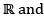α, β, α ≠ β are the only characteristic roots (eigenvalues) of A in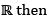the characteristic polynomail of A is

Solution:
QUESTION: 12

If A is symmetric matrix λ12,.... ,λn be the eigenvalues of A and a11,a22,.....,ann is the diagonal entries of A. Then which of the following is correct?

Solution:
QUESTION: 13

The minimal polynomial of the 3 x 3 real matrix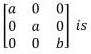Solution:
QUESTION: 14

A square matrix A is said to be idempotent if A2 = A. An idempotent matrix is non singular iff

Solution:
QUESTION: 15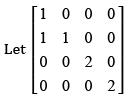be such that A has real eigenvalues then

Solution:
QUESTION: 16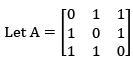then the eigenvalues of A are

Solution:
QUESTION: 17

Let A = [ajj] be an n x n matrix with real entries such that the sum of all the entries in each row is zero. Consider the following statements
(I) A is non-singular
(II) A is singular
(III) 0 is an eigenvalue of A

Which of the following is correct?

Solution:
QUESTION: 18

The minimal polynomial m(A) of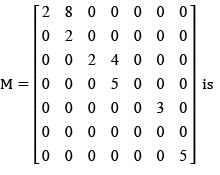Solution:
QUESTION: 19

Let A be a 2 x 2 real matrix of rank 1. If A is not diagonalizable then

Solution:
QUESTION: 20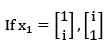are given vectors and A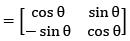and if P = [x1   x2] then P-1AP

Solution: